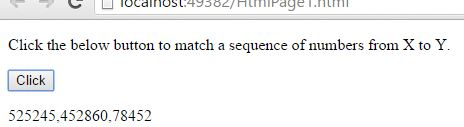# Matching sequence of X to Y characters in JavaScript

##### How to match any string that contains a sequence of numbers X to Y from specified digits in a string in JavaScript?

By using d{X,Y} quantifier we can match any string that contains a sequence of numbers X to Y from specified digits in a string.

NOTE:

• \d is the Metacharacter, which is used to find a digit.
• {X,Y} is the input value and it must be a number.
```<p>Click the below button to match a sequence of numbers from X to Y.</p>
<input type="button" value="Click" onclick="mySearch()" />
<p id="myId"></p>

<script>
function mySearch() {
var a = "525245, 45286012, 6215, 78452";
var b = /\d{5,6}/g;
var r = a.match(b);
document.getElementById("myId").innerHTML = r;
}
</script>
```

In the above code snippet we have given `Id` as "`myId`"to the second `<p>` element in the HTML code. There is a function mySearch() in the`<script>`block which is connected to the onclick of the HTML button and there is a string with value "525245, 45286012, 6215, 78452" to the variable `a`we need to match any string that contains a sequence of numbers X to Y, for that we are using `d{X,Y}` quantifier in variable `b`. `var b = /\d{5,6}/g` here `\d` is the metacharacter for finding a digit, {5,6} are the inputs of `{X,Y}`for matching a sequence of 5-digit to 6-digit numbers and `g` do the global search in a string, varaible `r` returns the matched sequence result. Onclick of the button "Click" in the HTML code fires the function mySearch() in the `<script>` block at the same time `d{X,Y}` quantifier matches any string that contains a sequence of X to Y (X=5 and Y=6) in a string with the help of global search `g` and gives the output.

OUTPUTViews: 2987 | Post Order: 147